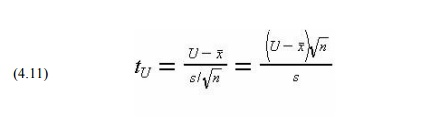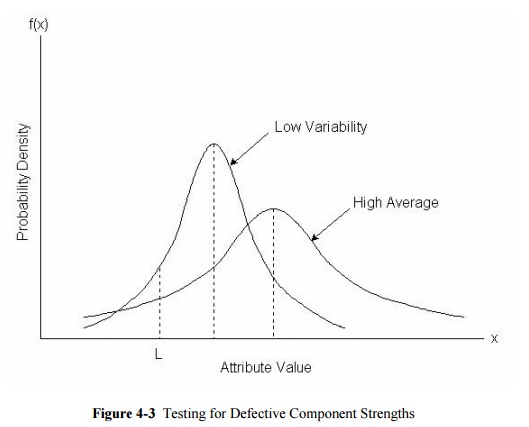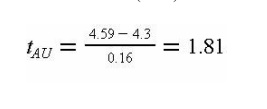Home | | Construction Planning and Scheduling | Statistical Quality Control with Sampling by Variables

# Statistical Quality Control with Sampling by VariablesMany work and material attributes possess continuous properties, such as strength, density or length. With the sampling by attributes procedure, a particular level of a variable quantity must be defined as acceptable quality.

Statistical Quality Control with Sampling by Variables

As described in the previous section, sampling by attributes is based on a classification of items as good or defective. Many work and material attributes possess continuous properties, such as strength, density or length. With the sampling by attributes procedure, a particular level of a variable quantity must be defined as acceptable quality. More generally, two items classified as good might have quite different strengths or other attributes. Intuitively, it seems reasonable that some "credit" should be provided for exceptionally good items in a sample. Sampling by variables was developed for application to continuously measurable quantities of this type. The procedure uses measured values of an attribute in a sample to determine the overall acceptability of a batch or lot. Sampling by variables has the advantage of using more information from tests since it is based on actual measured values rather than a simple classification. As a result, acceptance sampling by variables can be more efficient than sampling by attributes in the sense that fewer samples are required to obtain a desired level of quality control.

In applying sampling by variables, an acceptable lot quality can be defined with respect to an upper limit U, a lower limit L, or both. With these boundary conditions, an acceptable quality level can be defined as a maximum allowable fraction of defective items, M. In Figure 13-2, the probability distribution of item attribute x is illustrated. With an upper limit U, the fraction of defective items is equal to the area under the distribution function to the right of U (so that xU). This fraction of defective items would be compared to the allowable fraction M to determine the acceptability of a lot. With both a lower and an upper limit on acceptable quality, the fraction defective would be the fraction of items greater than the upper limit or less than the lower limit. Alternatively, the limits could be imposed upon the acceptable average level of the variable.In sampling by variables, the fraction of defective items is estimated by using measured values from a sample of items. As with sampling by attributes, the procedure assumes a random sample of a give size is obtained from a lot or batch. In the application of sampling by variables plans, the measured characteristic is virtually always assumed to be normally distributed as illustrated in Figure 13-2. The normal distribution is likely to be a reasonably good assumption for many measured characteristics such as material density or degree of soil compaction. The Central Limit Theorem provides a general support for the assumption: if the source of variations is a large number of small and independent random effects, then the resulting distribution of values will approximate the normal distribution. If the distribution of measured values is not likely to be approximately normal, then sampling by attributes should be adopted. Deviations from normal distributions may appear as skewed or non-symmetric distributions, or as distributions with fixed upper and lower limits.

The fraction of defective items in a sample or the chance that the population average has different values is estimated from two statistics obtained from the sample: the sample mean and standard deviation. Mathematically, let n be the number of items in the sample and xi, i = 1,2,3,...,n, be the

measured values of the variable characteristic x. Then an estimate of the overall population mean m  is the sample mean:Based on these two estimated parameters and the desired limits, the various fractions of interest for the population can be calculated.

The probability that the average value of a population is greater than a particular lower limit is calculated from the test statistic:which is t-distributed with n-1 degrees of freedom. If the population standard deviation is known in advance, then this known value is substituted for the estimate s and the resulting test statistic would be normally distributed. The t distribution is similar in appearance to a standard normal distribution, although the spread or variability in the function decreases as the degrees of freedom parameter increases. As the number of degrees of freedom becomes very large, the t-distribution coincides with the normal distribution.

With an upper limit, the calculations are similar, and the probability that the average value of a population is less than a particular upper limit can be calculated from the test statistic:With both upper and lower limits, the sum of the probabilities of being above the upper limit or below the lower limit can be calculated.

The calculations to estimate the fraction of items above an upper limit or below a lower limit are very

similar to those for the population average. The only difference is that the square root of the number of samples does not appear in the test statistic formulas:where tAL is the test statistic for all items with a lower limit and tAU is the test statistic for all items

with a upper limit. For example, the test statistic for items above an upper limit of 5.5 with= 4.0, s = 3.0, and n = 5 is tAU = (8.5 - 4.0)/3.0 = 1.5 with n - 1 = 4 degrees of freedom.

Instead of using sampling plans that specify an allowable fraction of defective items, it saves computations to simply write specifications in terms of the allowable test statistic values themselves. This procedure is equivalent to requiring that the sample average be at least a pre-specified number of standard deviations away from an upper or lower limit. For example, with= 4.0, U = 8.5, s = 3.0 and n = 41, the sample mean is only about (8.5 - 4.0)/3.0 = 1.5 standard deviations away from the upper limit.

To summarize, the application of sampling by variables requires the specification of a sample size, the relevant upper or limits, and either (1) the allowable fraction of items falling outside the designated limits or (2) the allowable probability that the population average falls outside the designated limit. Random samples are drawn from a pre-defined population and tested to obtained measured values of a variable attribute. From these measurements, the sample mean, standard deviation, and quality control test statistic are calculated. Finally, the test statistic is compared to the allowable trigger level and the lot is either accepted or rejected. It is also possible to apply sequential sampling in this procedure, so that a batch may be subjected to additional sampling and testing to further refine the test statistic values.

With sampling by variables, it is notable that a producer of material or work can adopt two general strategies for meeting the required specifications. First, a producer may insure that the average quality level is quite high, even if the variability among items is high. This strategy is illustrated in Figure 4-3 as a "high quality average" strategy. Second, a producer may meet a desired quality target by reducing the variability within each batch. In Figure 4-3, this is labeled the "low variability" strategy. In either case, a producer should maintain high standards to avoid rejection of a batch.Example 4-5: Testing for defective component strengths

Suppose that an inspector takes eight strength measurements with the following results:

4.3, 4.8, 4.6, 4.7, 4.4, 4.6, 4.7, 4.6

In this case, the sample mean and standard deviation can be calculated using Equations (13.8) and (13.9):

x = 1/8(4.3 + 4.8 + 4.6 + 4.7 + 4.4 + 4.6 + 4.7 + 4.6) = 4.59

s2=[1/(8-1)][(4.3 - 4.59) 2 + (4.8 - 4.59)2 + (4.6 - 4.59)2 + (4.7 - 4.59)2 + (4.4 - 4.59)2 + (4.6 - 4.59)2 + (4.7 - 4.59)2 + (4.6 - 4.59)2] = 0.16

The percentage of items below a lower quality limit of L = 4.3 is estimated from the test statistic tAL in Equation (13.12):Study Material, Lecturing Notes, Assignment, Reference, Wiki description explanation, brief detail
Civil : Construction Planning And Scheduling : Quality Control and Safety during Construction : Statistical Quality Control with Sampling by Variables |